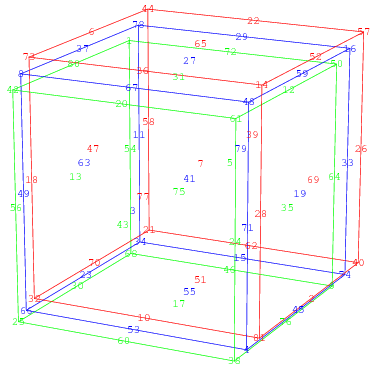### Features of the four dimensional magic hypercube

A hyper cube is a cube which has more than three dimensions. What?

A magic square is flat and that is two dimensional. A magic cube is three dimensional. But what is four dimensional? See below a four dimensional (hyper) cube (source: http://mathworld.wolfram.com/ MagicTesseract.html).A four dimensional 3x3x3x3 cube (n.b.: a 4D cube is also called a tesseract) consists of three 3x3x3 cubes (see above red, blew and green) which are put over each other. That gives besides rows and columns (= two dimensional) and pillars (= three dimensional), posts (= four dimensional). There are also space diagonals, but these are four (in stead of three) dimensional. It is easier to put the three 3x3x3 cubes next to (in stead of over) each other, so I can make all magic features clear:

 T1, level I T2, level I T3, level I a1 b1 c1 a1 b1 c1 a1 b1 c1 a2 b2 c2 a2 b2 c2 a2 b2 c2 a3 b3 c3 a3 b3 c3 a3 b3 c3 T1, level II T2, level II T3, level II a1 b1 c1 a1 b1 c1 a1 b1 c1 a2 b2 c2 a2 b2 c2 a2 b2 c2 a3 b3 c3 a3 b3 c3 a3 b3 c3 T1, level III T2, level III T3, level III a1 b1 c1 a1 b1 c1 a1 b1 c1 a2 b2 c2 a2 b2 c2 a2 b2 c2 a3 b3 c3 a3 b3 c3 a3 b3 c3

Simple magic cube (boundary magic features):

• all rows give magic sum (27x): a1 + b1 + c1 = a2 + b2 + c2 = a3 + b3 + c3
• all columns give magic sum (27x): a1 + a2 + a3 = b1 + b2 + b3 = c1 + c2 + c3
• all pillars give magic sum (81x): I a1+IIa1+III a1=Ia2+IIa2+IIIa2=...=Ic3+IIc3+IIIc3
• all posts give magic sum (81x): T1Ia1+T2Ia1+T3Ia1= ... = T1III c3+T2III c3+T3 III c3
• all 4 dimensional diagonals (8x) is 2 cells with same colour + middle cell (b2) give magic sum.

Magic cube, (pan)diagonal in levels (additional magic feature):

• all (pan)diagonals in all levels give magic sum: a1+b2+c3 = c1+b2+a3 = b1+c2+a3 = c1+a2+a3 = c2+b3+a1 = c3+b1+a2

Diagonal magic cube (additional magic features):

• All diagonals in levels give magic sum: a1 + b2 + c3 = c1 + b2 + a3
• All diagonals through levels in two directions give magic sum:                                      Ia/b/c1+IIa/b/c2+IIIa/b/c3=Ia1/2/3+IIb1/2/3+IIIc1/2/3 or T1a/b/c1+T2a/b/c2+T3a/b/c3=T1a1/2/3+T2b1/2/3+T3c1/2/3

Pandiagonal magic cube (additional magic features):

• All (pan)diagonals in all levels give magic sum: a1+b2+c3 = c1+b2+a3 = b1+c2+a3 = c1+a2+a3 = c2+b3+a1 = c3+b1+a2
• All diagonals through levels in two directions give magic sum:                                      Ia/b/c1+IIa/b/c2+IIIa/b/c3=Ia1/2/3+IIb1/2/3+IIIc1/2/3 or T1a/b/c1+T2a/b/c2+T3a/b/c3=T1a1/2/3+T2b1/2/3+T3c1/2/3
• All pandiagonals through levels in two directions give magic sum:                              Ia/b/c2+IIa/b/c3+IIIa/b/c1=Ib1/2/3+IIc1/2/3+IIIa1/2/3 or T1a/b/c2+T2a/b/c3+T3a/b/c1=T1b1/2/3+T2c1/2/3+T3a1/2/3

Pantriagonal magic cube (additional magic feature):

• All (pan)triagonals through the levels in two directions give magic sum:               e.g. Ia1+IIb2+IIIc3=Ic2+IIb3+IIIa1 or T1a1+T2b2+T3c3=T1c2+T2b3+T3a1